## Friday, August 26, 2016 ...//### The delirium over beryllium

Guest blog by Prof Flip Tanedo, a co-author of the first highlighted paper and the editor-in-chief of Particle Bites

 Click at the pirate flag above for a widget-free versionArticle: Particle Physics Models for the $$17\MeV$$ Anomaly in Beryllium Nuclear Decays
Authors: J.L. Feng, B. Fornal, I. Galon, S. Gardner, J. Smolinsky, T. M. P. Tait, F. Tanedo
Reference: arXiv:1608.03591 (Submitted to Phys. Rev. D)

Also featuring the results from:
• Gulyás et al., “A pair spectrometer for measuring multipolarities of energetic nuclear transitions” (description of detector; 1504.00489; NIM)
• Krasznahorkay et al., “Observation of Anomalous Internal Pair Creation in 8Be: A Possible Indication of a Light, Neutral Boson” (experimental result; 1504.01527; PRL version; note PRL version differs from arXiv)
• Feng et al., “Protophobic Fifth-Force Interpretation of the Observed Anomaly in 8Be Nuclear Transitions” (phenomenology; 1604.07411; PRL)
Recently there’s some press (see links below) regarding early hints of a new particle observed in a nuclear physics experiment. In this bite, we’ll summarize the result that has raised the eyebrows of some physicists, and the hackles of others.

A crash course on nuclear physics

Nuclei are bound states of protons and neutrons. They can have excited states analogous to the excited states of atoms, which are bound states of nuclei and electrons. The particular nucleus of interest is beryllium-8, which has four neutrons and four protons, which you may know from the triple alpha process. There are three nuclear states to be aware of: the ground state, the $$18.15\MeV$$ excited state, and the $$17.64\MeV$$ excited state.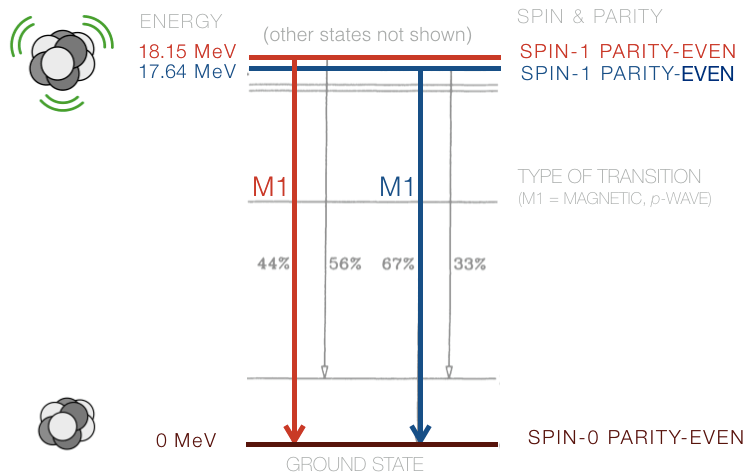Beryllium-8 excited nuclear states. The $$18.15\MeV$$ state (red) exhibits an anomaly. Both the $$18.15\MeV$$ and $$17.64\MeV$$ states decay to the ground through a magnetic, $$p$$-wave transition. Image adapted from Savage et al. (1987). Click at images to zoom in.

Most of the time the excited states fall apart into a lithium-7 nucleus and a proton. But sometimes, these excited states decay into the beryllium-8 ground state by emitting a photon (γ-ray). Even more rarely, these states can decay to the ground state by emitting an electron-positron pair from a virtual photon: this is called internal pair creation and it is these events that exhibit an anomaly.

The beryllium-8 anomaly

Physicists at the Atomki nuclear physics institute in Hungary were studying the nuclear decays of excited beryllium-8 nuclei. The team, led by Attila J. Krasznahorkay, produced beryllium excited states by bombarding a lithium-7 nucleus with protons.Beryllium-8 excited states are prepared by bombarding lithium-7 with protons.

The proton beam is tuned to very specific energies so that one can ‘tickle’ specific beryllium excited states. When the protons have around $$1.03\MeV$$ of kinetic energy, they excite lithium into the $$18.15\MeV$$ beryllium state. This has two important features:
1. Picking the proton energy allows one to only produce a specific excited state so one doesn’t have to worry about contamination from decays of other excited states.
2. Because the $$18.15\MeV$$ beryllium nucleus is produced at resonance, one has a very high yield of these excited states. This is very good when looking for very rare decay processes like internal pair creation.
What one expects is that most of the electron-positron pairs have small opening angle with a smoothly decreasing number as with larger opening angles.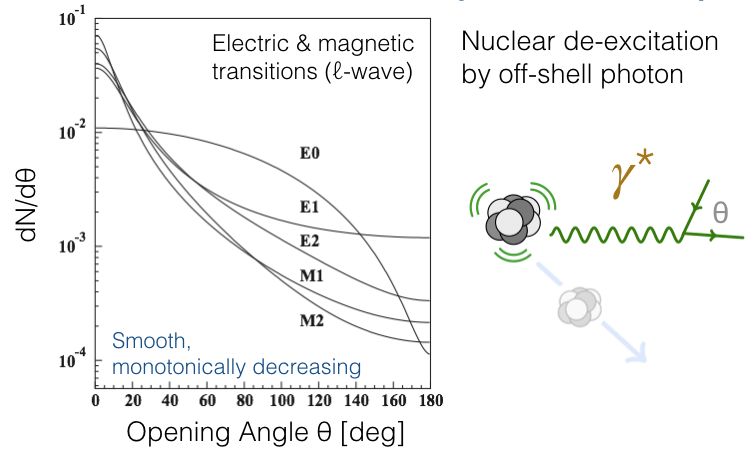Expected distribution of opening angles for ordinary internal pair creation events. Each line corresponds to nuclear transition that is electric (E) or magnetic (M) with a given orbital quantum number, $$\ell$$. The beryllium transitions that we’re interested in are mostly M1. Adapted from Gulyás et al. (1504.00489).

Instead, the Atomki team found an excess of events with large electron-positron opening angle. In fact, even more intriguing: the excess occurs around a particular opening angle (140 degrees) and forms a bump.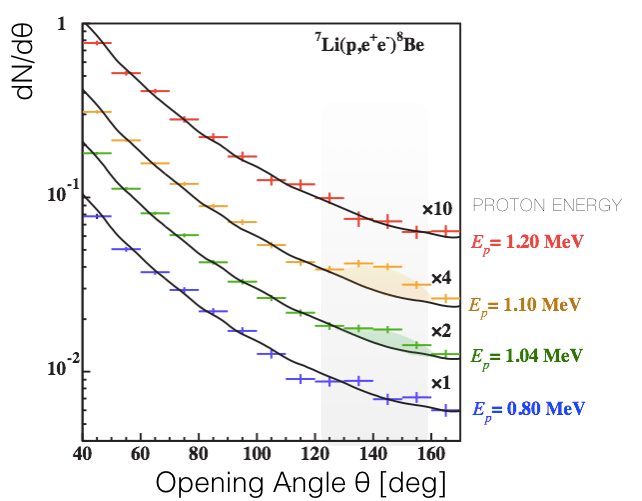Number of events ($${\rm d}N/{\rm d}\theta$$) for different electron-positron opening angles and plotted for different excitation energies ($$E_p$$). For $$E_p=1.10\MeV$$, there is a pronounced bump at 140 degrees which does not appear to be explainable from the ordinary internal pair conversion. This may be suggestive of a new particle. Adapted from Krasznahorkay et al., PRL 116, 042501.

Here’s why a bump is particularly interesting:
1. The distribution of ordinary internal pair creation events is smoothly decreasing and so this is very unlikely to produce a bump.
2. Bumps can be signs of new particles: if there is a new, light particle that can facilitate the decay, one would expect a bump at an opening angle that depends on the new particle mass.
Schematically, the new particle interpretation looks like this: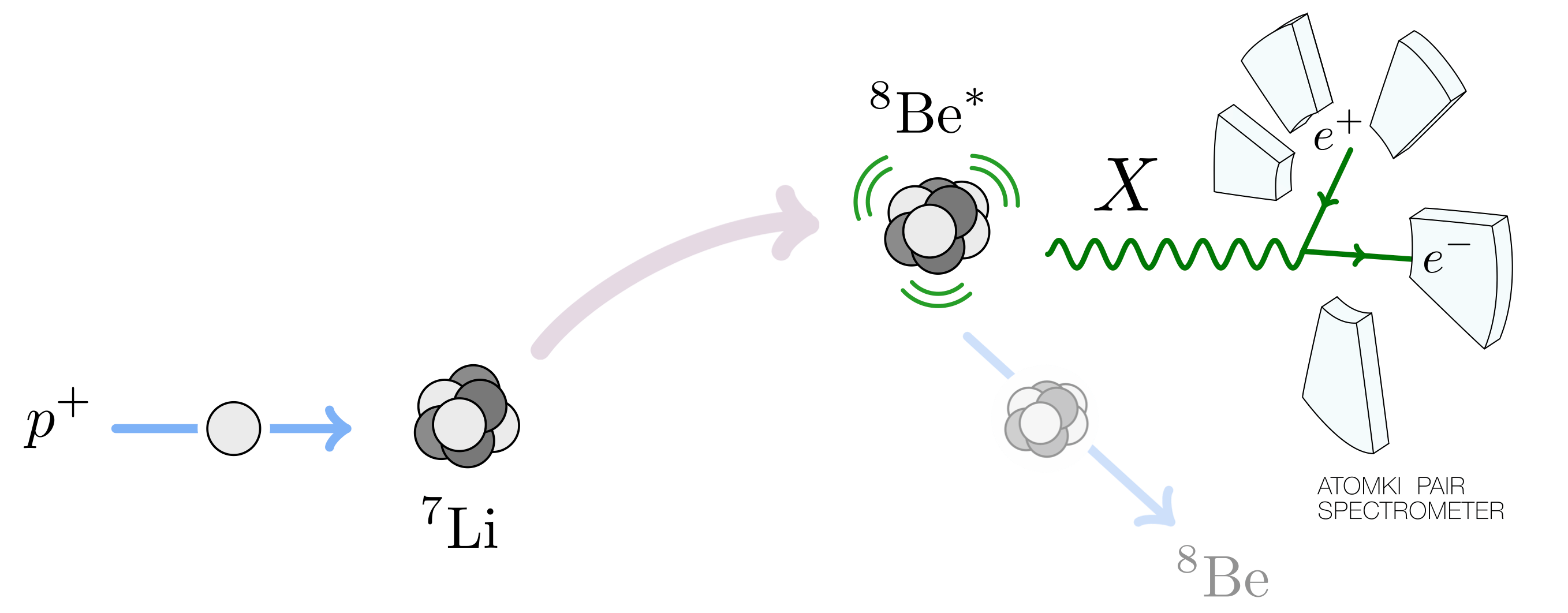Schematic of the Atomki experiment and new particle ($$X$$) interpretation of the anomalous events. In summary: protons of a specific energy bombard stationary lithium-7 nuclei and excite them to the $$18.15\MeV$$ beryllium-8 state. These decay into the beryllium-8 ground state. Some of these decays are mediated by the new $$X$$ particle, which then decays in to electron-positron pairs of a certain opening angle that are detected in the Atomki pair spectrometer detector. Image from 1608.03591.

As an exercise for those with a background in special relativity, one can use the relation $$(p_{e^+}+p_{e^-})^2 = m_X^2$$ to prove the result:$m_X^2 = \zav{ 1 - \zav{\frac{E_{e^+}\!-\!E_{e^-}}{E_{e^+}\!+\!E_{e^-}}}^{\!2} } (E_{e^+}\!+\!E_{e^-})^2 \sin^2 \frac\theta 2$ This relates the mass of the proposed new particle, $$X$$, to the opening angle $$\theta$$ and the energies $$E$$ of the electron and positron. The opening angle bump would then be interpreted as a new particle with mass of roughly $$17\MeV$$. To match the observed number of anomalous events, the rate at which the excited beryllium decays via the $$X$$ boson must be $$6\times 10^{-6}$$ times the rate at which it goes into a γ-ray.

The anomaly has a significance of 6.8σ. This means that it’s highly unlikely to be a statistical fluctuation, as the $$750\GeV$$ diphoton bump appears to have been. Indeed, the conservative bet would be some not-understood systematic effect, akin to the $$130\GeV$$ Fermi γ-ray line.

The beryllium that cried wolf?

Some physicists are concerned that beryllium may be the ‘boy that cried wolf,’ and point to papers by the late Fokke de Boer as early as 1996 and all the way to 2001. de Boer made strong claims about evidence for a new $$10\MeV$$ particle in the internal pair creation decays of the $$17.64\MeV$$ beryllium-8 excited state. These claims didn’t pan out, and in fact the instrumentation paper by the Atomki experiment rules out that original anomaly.

The proposed evidence for “de Boeron” is shown below: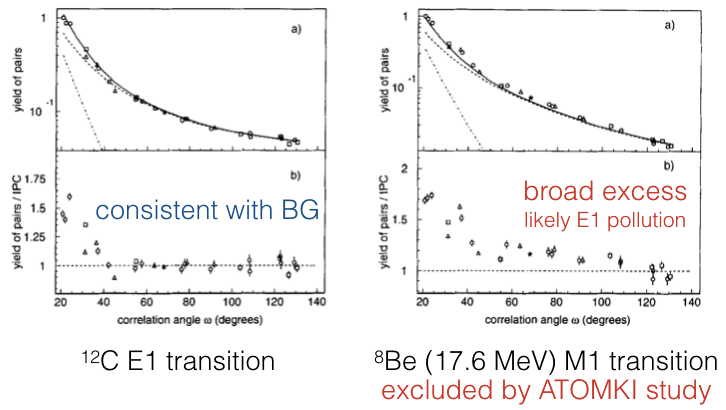The de Boer claim for a $$10\MeV$$ new particle. Left: distribution of opening angles for internal pair creation events in an E1 transition of carbon-12. This transition has similar energy splitting to the beryllium-8 $$17.64\MeV$$ transition and shows good agreement with the expectations; as shown by the flat “signal – background” on the bottom panel. Right: the same analysis for the M1 internal pair creation events from the $$17.64\MeV$$ beryllium-8 states. The “signal – background” now shows a broad excess across all opening angles. Adapted from de Boer et al. PLB 368, 235 (1996).

When the Atomki group studied the same $$17.64\MeV$$ transition, they found that a key background component—subdominant E1 decays from nearby excited states—dramatically improved the fit and were not included in the original de Boer analysis. This is the last nail in the coffin for the proposed $$10\MeV$$ “de Boeron.”

However, the Atomki group also highlight how their new anomaly in the $$18.15\MeV$$ state behaves differently. Unlike the broad excess in the de Boer result, the new excess is concentrated in a bump. There is no known way in which additional internal pair creation backgrounds can contribute to add a bump in the opening angle distribution; as noted above: all of these distributions are smoothly falling.

The Atomki group goes on to suggest that the new particle appears to fit the bill for a dark photon, a reasonably well-motivated copy of the ordinary photon that differs in its overall strength and having a non-zero ($$17\MeV$$?) mass.

Theory part 1: Not a dark photon

With the Atomki result was published and peer reviewed in Physics Review Letters, the game was afoot for theorists to understand how it would fit into a theoretical framework like the dark photon. A group from UC Irvine, University of Kentucky, and UC Riverside found that actually, dark photons have a hard time fitting the anomaly simultaneously with other experimental constraints. In the visual language of this recent ParticleBite, the situation was this: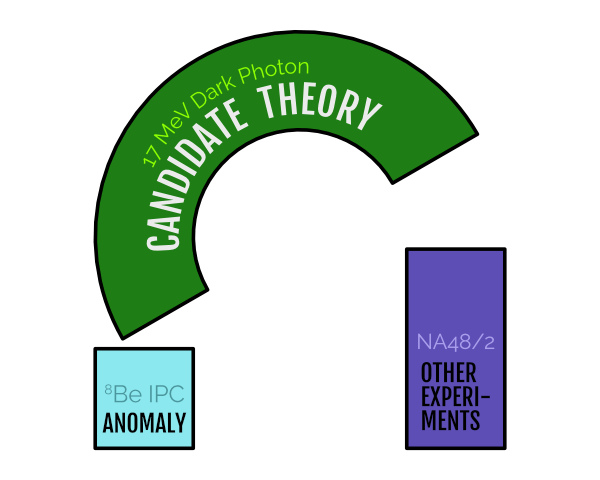It turns out that the minimal model of a dark photon cannot simultaneously explain the Atomki beryllium-8 anomaly without running afoul of other experimental constraints. Image adapted from this ParticleBite.

The main reason for this is that a dark photon with mass and interaction strength to fit the beryllium anomaly would necessarily have been seen by the NA48/2 experiment. This experiment looks for dark photons in the decay of neutral pions ($$\pi^0$$). These pions typically decay into two photons, but if there’s a $$17\MeV$$ dark photon around, some fraction of those decays would go into dark-photon — ordinary-photon pairs. The non-observation of these unique decays rules out the dark photon interpretation.

The theorists then decided to “break” the dark photon theory in order to try to make it fit. They generalized the types of interactions that a new photon-like particle, $$X$$, could have, allowing protons, for example, to have completely different charges than electrons rather than having exactly opposite charges. Doing this does gross violence to the theoretical consistency of a theory—but they goal was just to see what a new particle interpretation would have to look like. They found that if a new photon-like particle talked to neutrons but not protons—that is, the new force were protophobic—then a theory might hold together.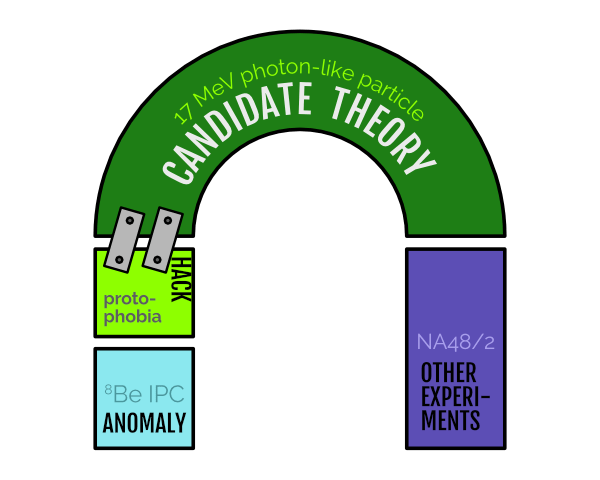Schematic description of how model-builders “hacked” the dark photon theory to fit both the beryllium anomaly while being consistent with other experiments. This hack isn’t pretty—and indeed, comes at the cost of potentially invalidating the mathematical consistency of the theory—but the exercise demonstrates the target for how to a complete theory might have to behave. Image adapted from this ParticleBite.

Theory appendix: pion-phobia is protophobia

Editor’s note: what follows is for readers with some physics background interested in a technical detail; others may skip this section.

How does a new particle that is allergic to protons avoid the neutral pion decay bounds from NA48/2? Pions decay into pairs of photons through the well-known triangle-diagrams of the axial anomaly. The decay into photon–dark-photon pairs proceed through similar diagrams. The goal is then to make sure that these diagrams cancel.

A cute way to look at this is to assume that at low energies, the relevant particles running in the loop aren’t quarks, but rather nucleons (protons and neutrons). In fact, since only the proton can talk to the photon, one only needs to consider proton loops. Thus if the new photon-like particle, $$X$$, doesn’t talk to protons, then there’s no diagram for the pion to decay into $$\gamma X$$. This would be great if the story weren’t completely wrong.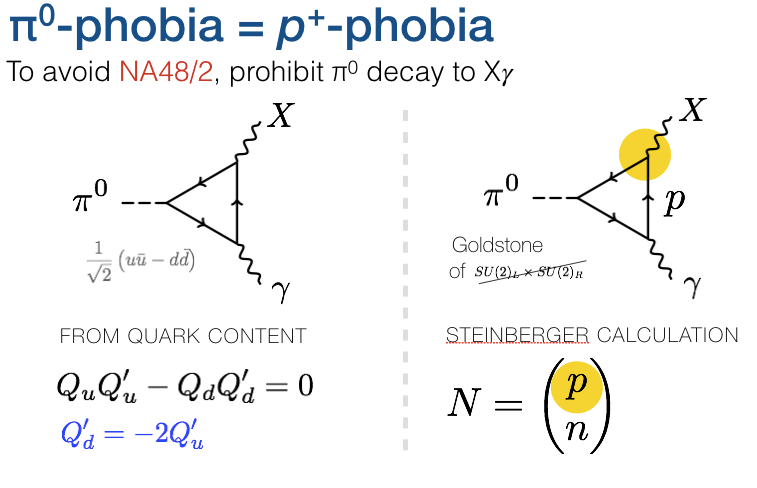Avoiding NA48/2 bounds requires that the new particle, $$X$$, is pion-phobic. It turns out that this is equivalent to $$X$$ being protophobic. The correct way to see this is on the left, making sure that the contribution of up-quark loops cancels the contribution from down-quark loops. A slick (but naively completely wrong) calculation is on the right, arguing that effectively only protons run in the loop.

The correct way of seeing this is to treat the pion as a quantum superposition of an up–anti-up and down–anti-down bound state, and then make sure that the $$X$$ charges are such that the contributions of the two states cancel. The resulting charges turn out to be protophobic.

The fact that the “proton-in-the-loop” picture gives the correct charges, however, is no coincidence. Indeed, this was precisely how Jack Steinberger calculated the correct pion decay rate. The key here is whether one treats the quarks/nucleons linearly or non-linearly in chiral perturbation theory. The relation to the Wess-Zumino-Witten term—which is what really encodes the low-energy interaction—is carefully explained in chapter 6a.2 of Georgi’s revised Weak Interactions.

Theory part 2: Not a spin-0 particle

The above considerations focus on a new particle with the same spin and parity as a photon (spin-1, parity odd). Another result of the UCI study was a systematic exploration of other possibilities. They found that the beryllium anomaly could not be consistent with spin-0 particles. For a parity-odd, spin-0 particle, one cannot simultaneously conserve angular momentum and parity in the decay of the excited beryllium-8 state. (Parity violating effects are negligible at these energies.)Parity and angular momentum conservation prohibit a “dark Higgs” (parity even scalar) from mediating the anomaly.

For a parity-odd pseudoscalar, the bounds on axion-like particles at $$20\MeV$$ suffocate any reasonable coupling. Measured in terms of the pseudoscalar–photon–photon coupling (which has dimensions of inverse $${\rm GeV}$$), this interaction is ruled out down to the inverse Planck scale.Bounds on axion-like particles exclude a $$20\MeV$$ pseudoscalar with couplings to photons stronger than the inverse Planck scale. Adapted from 1205.2671 and 1512.03069.

• Dark Z-bosons, cousins of the dark photon with spin-1 but indeterminate parity. This is very constrained by atomic parity violation.
• Axial vectors, spin-1 bosons with positive parity. These remain a theoretical possibility, though their unknown nuclear matrix elements make it difficult to write a predictive model. (See section II.D of 1608.03591.)
Theory part 3: Nuclear input

The plot thickens when once also includes results from nuclear theory. Recent results from Saori Pastore, Bob Wiringa, and collaborators point out a very important fact: the $$18.15\MeV$$ beryllium-8 state that exhibits the anomaly and the $$17.64\MeV$$ state which does not are actually closely related.

Recall (e.g. from the first figure at the top) that both the $$18.15\MeV$$ and $$17.64\MeV$$ states are both spin-1 and parity-even. They differ in mass and in one other key aspect: the $$17.64\MeV$$ state carries isospin charge, while the $$18.15\MeV$$ state and ground state do not.

Isospin is the nuclear symmetry that relates protons to neutrons and is tied to electroweak symmetry in the full Standard Model. At nuclear energies, isospin charge is approximately conserved. This brings us to the following puzzle:
If the new particle has mass around $$17\MeV$$, why do we see its effects in the $$18.15\MeV$$ state but not the $$17.64\MeV$$ state?
Naively, if the new particle emitted, $$X$$, carries no isospin charge, then isospin conservation prohibits the decay of the $$17.64\MeV$$ state through emission of an $$X$$ boson. However, the Pastore et al. result tells us that actually, the isospin-neutral and isospin-charged states mix quantum mechanically so that the observed $$18.15$$ and $$17.64\MeV$$ states are mixtures of iso-neutral and iso-charged states. In fact, this mixing is actually rather large, with mixing angle of around 10 degrees!

The result of this is that one cannot invoke isospin conservation to explain the non-observation of an anomaly in the $$17.64\MeV$$ state. In fact, the only way to avoid this is to assume that the mass of the $$X$$ particle is on the heavier side of the experimentally allowed range. The rate for $$X$$ emission goes like the 3-momentum cubed (see section II.E of 1608.03591), so a small increase in the mass can suppresses the rate of $$X$$ emission by the lighter state by a lot.

The UCI collaboration of theorists went further and extended the Pastore et al. analysis to include a phenomenological parameterization of explicit isospin violation. Independent of the Atomki anomaly, they found that including isospin violation improved the fit for the $$18.15\MeV$$ and $$17.64\MeV$$ electromagnetic decay widths within the Pastore et al. formalism. The results of including all of the isospin effects end up changing the particle physics story of the Atomki anomaly significantly:The rate of $$X$$ emission (colored contours) as a function of the $$X$$ particle’s couplings to protons (horizontal axis) versus neutrons (vertical axis). The best fit for a $$16.7\MeV$$ new particle is the dashed line in the teal region. The vertical band is the region allowed by the NA48/2 experiment. Solid lines show the dark photon and protophobic limits. Left: the case for perfect (unrealistic) isospin. Right: the case when isospin mixing and explicit violation are included. Observe that incorporating realistic isospin happens to have only a modest effect in the protophobic region. Figure from 1608.03591.

The results of the nuclear analysis are thus that:
1. An interpretation of the Atomki anomaly in terms of a new particle tends to push for a slightly heavier $$X$$ mass than the reported best fit. (Remark: the Atomki paper does not do a combined fit for the mass and coupling nor does it report the difficult-to-quantify systematic errors associated with the fit. This information is important for understanding the extent to which the $$X$$ mass can be pushed to be heavier.)
2. The effects of isospin mixing and violation are important to include; especially as one drifts away from the purely protophobic limit.
Theory part 4: towards a complete theory

The theoretical structure presented above gives a framework to do phenomenology: fitting the observed anomaly to a particle physics model and then comparing that model to other experiments. This, however, doesn’t guarantee that a nice—or even self-consistent—theory exists that can stretch over the scaffolding.

Indeed, a few challenges appear:
• The isospin mixing discussed above means the $$X$$ mass must be pushed to the heavier values allowed by the Atomki observation.
• The “protophobic” limit is not obviously anomaly-free: simply asserting that known particles have arbitrary charges does not generically produce a mathematically self-consistent theory.
• Atomic parity violation constraints require that the $$X$$ couple in the same way to left-handed and right-handed matter. The left-handed coupling implies that $$X$$ must also talk to neutrinos: these open up new experimental constraints.
The Irvine/Kentucky/Riverside collaboration first note the need for a careful experimental analysis of the actual mass ranges allowed by the Atomki observation, treating the new particle mass and coupling as simultaneously free parameters in the fit.

Next, they observe that protophobic couplings can be relatively natural. Indeed: the Standard Model Z-boson is approximately protophobic at low energies—a fact well known to those hunting for dark matter with direct detection experiments. For exotic new physics, one can engineer protophobia through a phenomenon called kinetic mixing where two force particles mix into one another. A tuned admixture of electric charge and baryon number, ($$Q-B$$), is protophobic.

Baryon number, however, is an anomalous global symmetry—this means that one has to work hard to make a baryon-boson that mixes with the photon (see 1304.0576 and 1409.8165 for examples). Another alternative is if the photon kinetically mixes with not baryon number, but the anomaly-free combination of “baryon-minus-lepton number,” $$Q-(B-L)$$. This then forces one to apply additional model-building modules to deal with the neutrino interactions that come along with this scenario.

In the language of the ‘model building blocks’ above, result of this process looks schematically like this:A complete theory is completely mathematically self-consistent and satisfies existing constraints. The additional bells and whistles required for consistency make additional predictions for experimental searches. Pieces of the theory can sometimes be used to address other anomalies.

The theory collaboration presented examples of the two cases, and point out how the additional ‘bells and whistles’ required may tie to additional experimental handles to test these hypotheses. These are simple existence proofs for how complete models may be constructed.

What’s next?

We have delved rather deeply into the theoretical considerations of the Atomki anomaly. The analysis revealed some unexpected features with the types of new particles that could explain the anomaly (dark photon-like, but not exactly a dark photon), the role of nuclear effects (isospin mixing and breaking), and the kinds of features a complete theory needs to have to fit everything (be careful with anomalies and neutrinos). The single most important next step, however, is and has always been experimental verification of the result.

While the Atomki experiment continues to run with an upgraded detector, what’s really exciting is that a swath of experiments that are either ongoing or in construction will be able to probe the exact interactions required by the new particle interpretation of the anomaly. This means that the result can be independently verified or excluded within a few years. A selection of upcoming experiments is highlighted in section IX of 1608.03591:Other experiments that can probe the new particle interpretation of the Atomki anomaly. The horizontal axis is the new particle mass, the vertical axis is its coupling to electrons (normalized to the electric charge). The dark blue band is the target region for the Atomki anomaly. Figure from 1608.03591; assuming 100% branching ratio to electrons.

We highlight one particularly interesting search: recently a joint team of theorists and experimentalists at MIT proposed a way for the LHCb experiment to search for dark photon-like particles with masses and interaction strengths that were previously unexplored. The proposal makes use of the LHCb’s ability to pinpoint the production position of charged particle pairs and the copious amounts of D-mesons produced at Run 3 of the LHC. As seen in the figure above, the LHCb reach with this search thoroughly covers the Atomki anomaly region.

Implications

So where we stand is this:
• There is an unexpected result in a nuclear experiment that may be interpreted as a sign for new physics.
• The next steps in this story are independent experimental cross-checks; the threshold for a ‘discovery’ is if another experiment can verify these results.
• Meanwhile, a theoretical framework for understanding the results in terms of a new particle has been built and is ready-and-waiting. Some of the results of this analysis are important for faithful interpretation of the experimental results.
What if it’s nothing?

This is the conservative take—and indeed, we may well find that in a few years, the possibility that Atomki was observing a new particle will be completely dead. Or perhaps a source of systematic error will be identified and the bump will go away. That’s part of doing science.

Meanwhile, there are some important take-aways in this scenario. First is the reminder that the search for light, weakly coupled particles is an important frontier in particle physics. Second, for this particular anomaly, there are some neat take aways such as a demonstration of how effective field theory can be applied to nuclear physics (see e.g. chapter 3.1.2 of the new book by Petrov and Blechman, Amazon) and how tweaking our models of new particles can avoid troublesome experimental bounds. Finally, it’s a nice example of how particle physics and nuclear physics are not-too-distant cousins and how progress can be made in particle–nuclear collaborations—one of the Irvine group authors (Susan Gardner) is a bona fide nuclear theorist who was on sabbatical from the University of Kentucky.

What if it’s real?

This is a big “what if.” On the other hand, a 6.8σ effect is not a statistical fluctuation and there is no known nuclear physics to produce a new-particle-like bump given the analysis presented by the Atomki experimentalists.

The threshold for “real” is independent verification. If other experiments can confirm the anomaly, then this could be a huge step in our quest to go beyond the Standard Model. While this type of particle is unlikely to help with the Hierarchy problem of the Higgs mass, it could be a sign for other kinds of new physics. One example is the grand unification of the electroweak and strong forces; some of the ways in which these forces unify imply the existence of an additional force particle that may be light and may even have the types of couplings suggested by the anomaly.

Could it be related to other anomalies?

The Atomki anomaly isn’t the first particle physics curiosity to show up at the $${\rm MeV}$$ scale. While none of these other anomalies are necessarily related to the type of particle required for the Atomki result (they may not even be compatible!), it is helpful to remember that the $${\rm MeV}$$ scale may still have surprises in store for us.
• The KTeV anomaly: The rate at which neutral pions decay into electron-positron pairs appears to be off from the expectations based on chiral perturbation theory. In 0712.0007, a group of theorists found that this discrepancy could be fit to a new particle with axial couplings. If one fixes the mass of the proposed particle to be $$20\MeV$$, the resulting couplings happen to be in the same ballpark as those required for the Atomki anomaly. The important caveat here is that parameters for an axial vector to fit the Atomki anomaly are unknown, and mixed vector–axial states are severely constrained by atomic parity violation.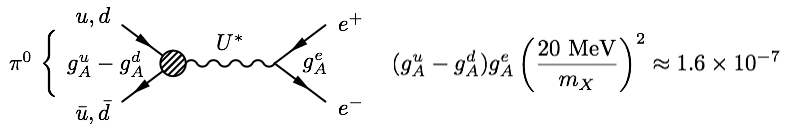The KTeV anomaly interpreted as a new particle, $$U$$. From 0712.0007.
• The anomalous magnetic moment of the muon and the cosmic lithium problem: much of the progress in the field of light, weakly coupled forces comes from Maxim Pospelov. The anomalous magnetic moment of the muon, $$(g-2)_\mu$$, has a long-standing discrepancy from the Standard Model (see e.g. this blog post). While this may come from an error in the very, very intricate calculation and the subtle ways in which experimental data feed into it, Pospelov (and also Fayet) noted that the shift may come from a light (in the $$10$$s of $${\rm MeV}$$ range!), weakly coupled new particle like a dark photon. Similarly, Pospelov and collaborators showed that a new light particle in the $$1$$-$$20\MeV$$ range may help explain another longstanding mystery: the surprising lack of lithium in the universe (APS Physics synopsis).
• The Proton Radius Problem: the charge radius of the proton appears to be smaller than expected when measured using the Lamb shift of muonic hydrogen versus electron scattering experiments. See this ParticleBite summary, and this recent review. Some attempts to explain this discrepancy have involved $${\rm MeV}$$-scale new particles, though the endeavor is difficult. There’s been some renewed popular interest after a new result using deuterium confirmed the discrepancy. However, there was a report that a result at the proton radius problem conference in Trento suggests that the 2S-4P determination of the Rydberg constant may solve the puzzle (though discrepant with other Rydberg measurements). [Those slides do not appear to be public.]
Could it be related to dark matter?

A lot of recent progress in dark matter has revolved around the possibility that in addition to dark matter, there may be additional light particles that mediate interactions between dark matter and the Standard Model. If these particles are light enough, they can change the way that we expect to find dark matter in sometimes surprising ways. One interesting avenue is called self-interacting dark matter and is based on the observation that these light force carriers can deform the dark matter distribution in galaxies in ways that seem to fit astronomical observations. A $$20\MeV$$ dark photon-like particle even fits the profile of what’s required by the self-interacting dark matter paradigm, though it is very difficult to make such a particle consistent with both the Atomki anomaly and the constraints from direct detection.

Should I be excited?

Given all of the caveats listed above, some feel that it is too early to be in “drop everything, this is new physics” mode. Others may take this as a hint that’s worth exploring further—as has been done for many anomalies in the recent past. For researchers, it is prudent to be cautious, and it is paramount to be careful; but so long as one does both, then being excited about a new possibility is part what makes our job fun.

For the general public, the tentative hopes of new physics that pop up—whether it’s the Atomki anomaly, or the $$750\GeV$$ diphoton bump, a $${\rm GeV}$$ bump from the galactic center, γ-ray lines at $$3.5\keV$$ and $$130\GeV$$, or penguins at LHCb—these are the signs that we’re making use of all of the data available to search for new physics. Sometimes these hopes fizzle away, often they leave behind useful lessons about physics and directions forward. Maybe one of these days an anomaly will stick and show us the way forward.